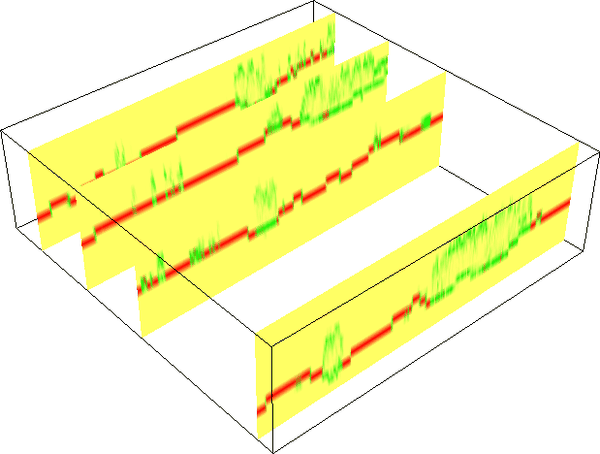Note: A new GRASS GIS stable version has been released: GRASS GIS 7.6, available here.
Updated manual page: here

## NAME

r3.in.lidar - Creates a 3D raster map from LAS LiDAR points

## KEYWORDS

3D raster, import, LIDAR

## SYNOPSIS

r3.in.lidar
r3.in.lidar --help
r3.in.lidar [-dv] input=name n=name sum=name mean=name proportional_n=name proportional_sum=name [return_filter=string] [class_filter=integer[,integer,...]] [base_raster=name] [--overwrite] [--help] [--verbose] [--quiet] [--ui]

### Flags:

-d
Use base raster actual resolution instead of computational region
-v
Use only valid points
Points invalid according to APSRS LAS specification will be filtered out
--overwrite
Allow output files to overwrite existing files
--help
Print usage summary
--verbose
Verbose module output
--quiet
Quiet module output
--ui
Force launching GUI dialog

### Parameters:

input=name [required]
LAS input file
LiDAR input file in LAS format (*.las or *.laz)
n=name [required]
Count of points per cell
Name for output 3D raster map
sum=name [required]
Sum of values of point intensities per cell
Name for output 3D raster map
mean=name [required]
Mean of point intensities per cell
Name for output 3D raster map
proportional_n=name [required]
3D raster map of proportional point count
Point count per 3D cell divided by point count per vertical column
proportional_sum=name [required]
3D raster map of proportional sum of values
Sum of values per 3D cell divided by sum of values per vertical column
return_filter=string
Only import points of selected return type
If not specified, all points are imported
Options: first, last, mid
class_filter=integer[,integer,...]
Only import points of selected class(es)
Input is comma separated integers. If not specified, all points are imported.
base_raster=name
Subtract raster values from the z coordinates
The scale for z is applied beforehand, the filter afterwards

## DESCRIPTIONFigure: Proportional count of points per 3D cell. When 50% of all points in a vertical column fall into a given 3D cell, the value is 0.5. Here, the green color was assigned to 0.5, red to 1 and yellow to 0. The figure shows vertical slices and green color indicates high vegetation while red color indicates bare ground.

## NOTES

• This module is highly experimental. Don't rely on its functionality or interface. Please report issues on the mailing list or in the bug tracker.
• No projection check or reprojection is performed, make sure you are using data in the right projection for your GRASS Location.
• Selection of points according to return or class is not yet supported.
• All outputs are currently mandatory.
• Some temporary maps are created but not cleaned up.
• Expects points to have intensity (random result for related outputs when they don't).

## EXAMPLES

Set the region according to a 2D raster and adding 3D minimum (bottom), maximum (top) and vertical (top-bottom) resolution.
```g.region rast=secref b=80 t=160 tbres=5 -p3
```
Now, r3.in.lidar will create the 3D raster of the size given by the computation region:
```r3.in.lidar input=points.las n=points_n sum=points_sum \
mean=points_mean proportional_n=points_n_prop \
proportional_sum=points_sum_prop
```

### Point density vertical structure reduced to the terrain

Create ground raster:
```r.in.lidar input=points.las output=ground method=mean class_filter=2
```
Set vertical extent of computational region to (relative) coordinates above ground:
```g.region rast=secref b=0 t=47 -p3
```
Compute point density:
```r3.in.lidar input=points.las n=points_n sum=points_sum \
mean=points_mean proportional_n=points_n_prop \
proportional_sum=points_sum_prop \
base_raster=ground
```

## SEE ALSO

r3.in.xyz, r.in.lidar, v.in.lidar, r.to.rast3, r3.mapcalc, g.region

## AUTHOR

Vaclav Petras, NCSU GeoForAll Lab

Last changed: \$Date: 2016-08-22 19:07:22 -0700 (Mon, 22 Aug 2016) \$

## SOURCE CODE

Available at: r3.in.lidar source code (history)

Note: A new GRASS GIS stable version has been released: GRASS GIS 7.6, available here.
Updated manual page: here

© 2003-2019 GRASS Development Team, GRASS GIS 7.2.4svn Reference Manual